# Geometry in College Preparatory Math: Help and Review Chapter Exam

Exam Instructions:

Choose your answers to the questions and click 'Next' to see the next set of questions. You can skip questions if you would like and come back to them later with the yellow "Go To First Skipped Question" button. When you have completed the practice exam, a green submit button will appear. Click it to see your results. Good luck!

### Page 1

#### Question 1 1. What is the relationship between Angle 2 and Angle 7?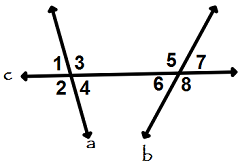#### Question 5 5. Given the triangle PQE, calculate the measure of Angle Q.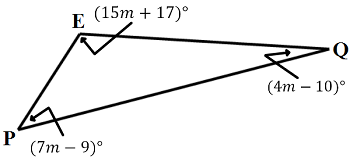### Page 2

#### Question 6 6. What is the area of ABCDE, as shown in the diagram?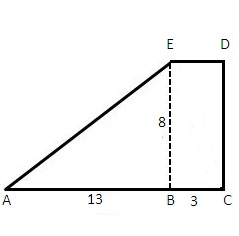#### Question 7 7. Which two lines are parallel?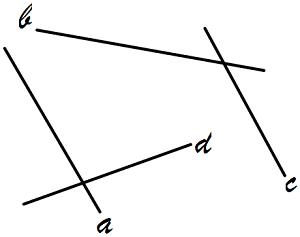#### Question 8 8. Consider the figure below, where Angle 4 = 12m - 4 Angle 5 = 10m + 20 Calculate the measure of Angle 5.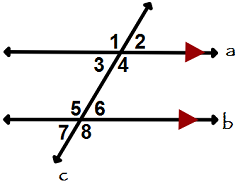#### Question 10 10. What is the line, as shown in the picture, called?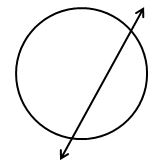### Page 3

#### Question 11 11. What is the area of the pictured triangle?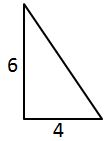#### Question 12 12. What is the relationship between Angle 1 and Angle 7?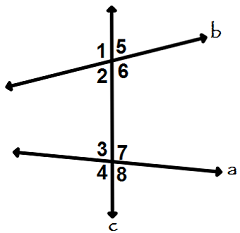#### Question 13 13. What type of triangle is pictured?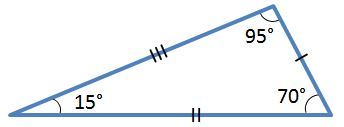### Page 4

#### Question 16 16. Josh claims that these triangles are similar by SSS. Do you agree or disagree with Josh?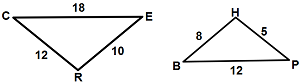#### Question 20 20. In the figure below, Angle 7 = 15x and Angle 10 = 9x + 30. Determine the measure of Angle 10.### Page 5

#### Question 21 21. Is triangle ABC similar to triangle DEF?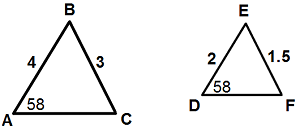#### Question 23 23. Given triangles DAR and KMR. What is the value of y?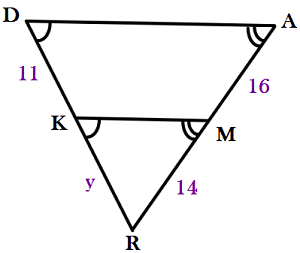#### Question 25 25. In the right triangle pictured below, what is the value of angle x?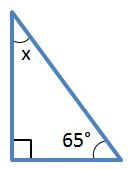### Page 6

#### Question 26 26. What is the perimeter of the pictured square?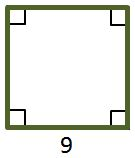#### Question 27 27. In the figure below, Angle 13 = 10y and Angle 11 = 3y + 11. Determine the measure of Angle 16.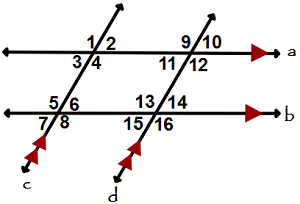#### Question 30 30. What is the relationship between Angle 4 and Angle 1?#### Geometry in College Preparatory Math: Help and Review Chapter Exam Instructions

Choose your answers to the questions and click 'Next' to see the next set of questions. You can skip questions if you would like and come back to them later with the yellow "Go To First Skipped Question" button. When you have completed the practice exam, a green submit button will appear. Click it to see your results. Good luck!

Support